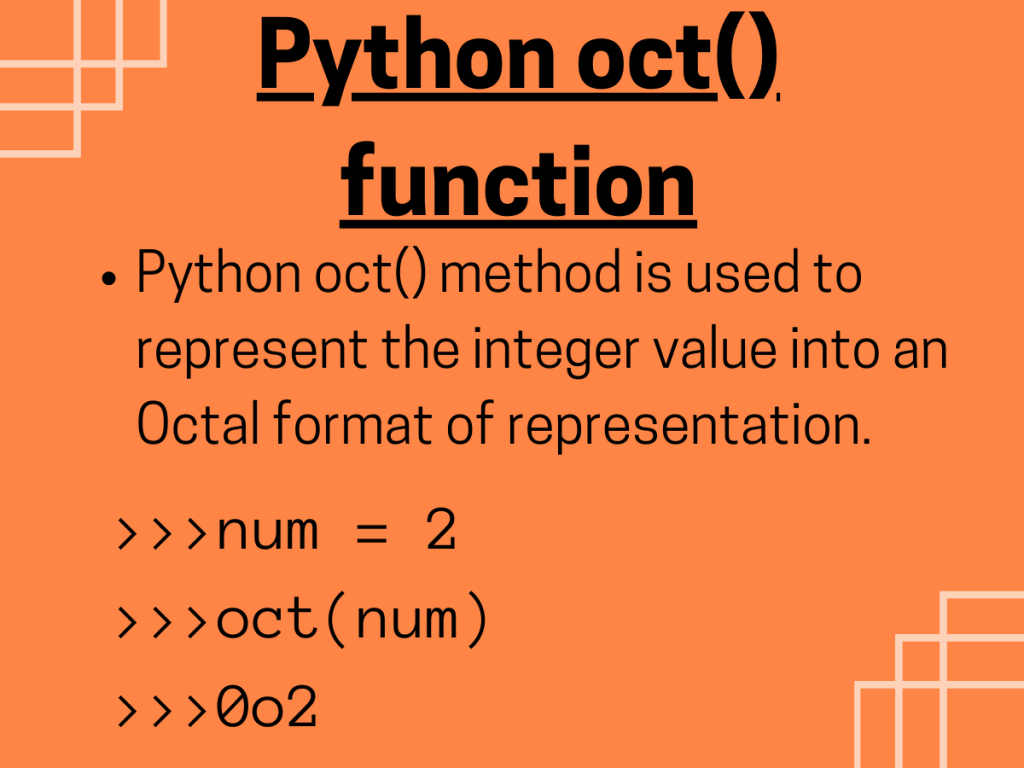# What is Python oct() function?Hey, folks! In this article, we will be focusing on the Octal representation of an integer value using Python oct() function.

## Getting started with Python oct() function

Python has various in-built functions to manipulate and operate over data values. `Python oct() method` is used to represent the integer value into an Octal format of representation.

Syntax:

```oct(number)
```
• `number`: The integer value that has to be passed to the function. It can be a decimal, binary or hexadecimal value.

Example:

```dec_num = 2
print("Octal representation of decimal number:", oct(dec_num))
bin_num = 0b10
print("Octal representation of binary number:", oct(bin_num))
hex_num = 0x17
print("Octal representation of decimal number:", oct(hex_num))
```

Output:

```Octal representation of decimal number: 0o2
Octal representation of binary number: 0o2
Octal representation of decimal number: 0o27
```

### Errors and Exceptions with Python oct() function

If a float type value is passed to Python oct() function, it raises a `TypeError` exception i.e. oct() function accepts only integer values as parameter.

Example:

```dec_num = 2.4
print("Octal representation of decimal number:", oct(dec_num))
```

Output:

```TypeError                                 Traceback (most recent call last)
<ipython-input-3-75c901e342e0> in <module>
1 dec_num = 2.4
----> 2 print("Octal representation of decimal number:", oct(dec_num))

TypeError: 'float' object cannot be interpreted as an integer

```

## Octal representation of elements of an array in NumPy module

The elements contained in a data structure such as NumPy arrays, lists, etc can be converted to its Octal forming using the built-in function of NumPy.

The `numpy.base_repr() function` is used to convert every element of the array to octal form in an element wise fashion.

Syntax:

```numpy.base_repr(number, base, padding)
```
• `number`: The element of the array whose octal format has to represented.
• `base`: The value which represents the resultant number system of the value to be converted. For octal representation, we need to place base = 8.
• `padding`: The number of zeros to be added to the left axis of the resultant number.

Example:

```import numpy as N
arr = [23,15,36,20]
res_arr = N.base_repr(arr,base = 8)
print("The Octal representation of",arr,":",res_arr)
```

Output:

```The Octal representation of 23 : 27
```

## Octal representation of data values using Pandas module

Python Pandas module is used to frame the elements in the form of DataFrames and operates over datasets. Python `apply(oct) function` can be used to represent the integer data values of the dataset into their Octal format.

Syntax:

```DataFrame.apply(oct)
```

Example:

```import pandas as pd
dec_data=pd.DataFrame({ 'Decimal-values':[20,32,7,23] })
oct_data=dec_data['Decimal-values'].apply(oct)
print(oct_data)
```

Output:

```0    0o24
1    0o40
2     0o7
3    0o27
Name: Decimal-values, dtype: object
```

## Conclusion

Thus, in this article, we have understood the way of representing the integer values into their Octal format using Python oct() function.

## References

• Python oct() function — JournalDev